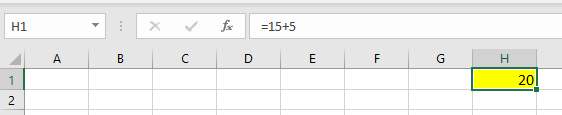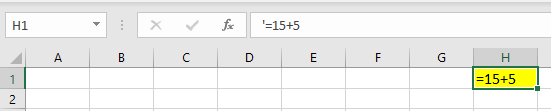What happens when you put single inverted comma before a formula in Excel?
Rajesh Pathak
Let's say you have this formula in cell H1
=15+5

Formula bar will show the formula and cell H1 will show the result 20What if you put a single inverted comma just before = sign in cell H1? You will convert the formula into text. Formula bar will show the single inverted comma that you have placed before = sign but cell H1 will show =15+5Also visit
Homepage​​​
MS EXCEL TRAINING​​​